Question

# We have the following sequence of instructions in MIPS lw \$t4, 4(\$s1) or \$t1, \$t2, \$t3...

We have the following sequence of instructions in MIPS

lw \$t4, 4(\$s1)

or \$t1, \$t2, \$t3

or \$t2, \$t1, \$t4

or \$t1, \$t1, \$t2

1) Indicate any hazards and what the hazard types are.

2) Assume there is no forwarding in this pipelined processor and each stage takes 1 cycle. Draw the pipeline chart and calculate how many cycles are consumed

3) Assume there is forwarding in this pipelined processor and each stage takes 1 cycle. Draw the pipeline chart and calculate how many cycles are consumed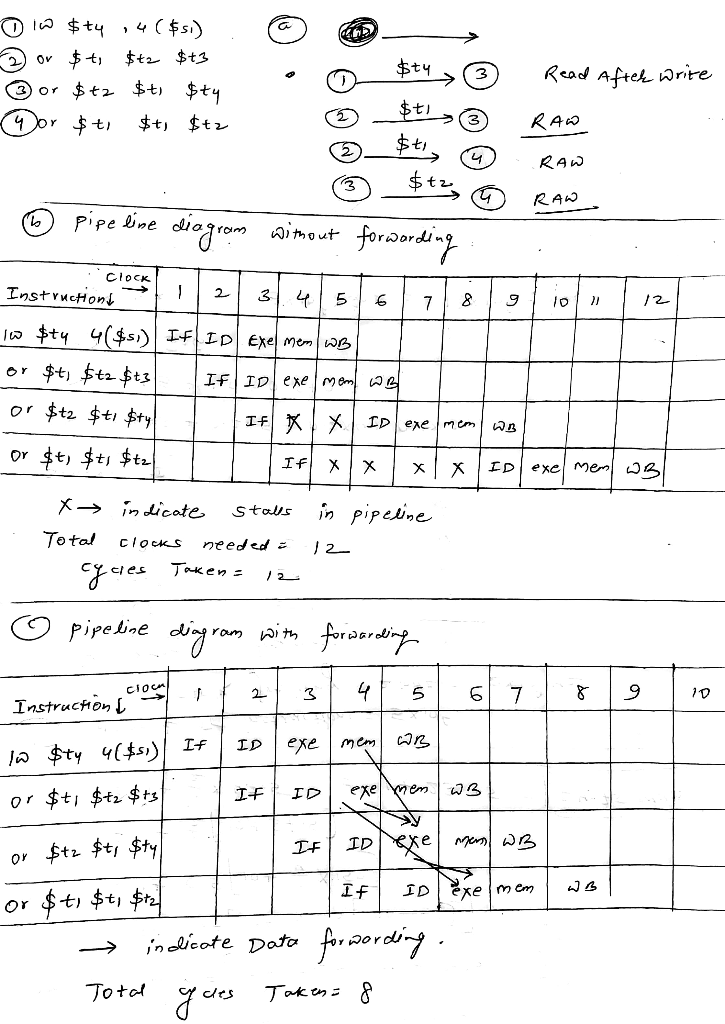#### Earn Coins

Coins can be redeemed for fabulous gifts.

Similar Homework Help Questions
• ### Given the following sequence of instructions: lw \$s2, 0(\$s1) //1 lw \$s1, 40(\$s3) //2 sub \$s3,...

Given the following sequence of instructions: lw \$s2, 0(\$s1) //1 lw \$s1, 40(\$s3) //2 sub \$s3, \$s1, \$s2 //3 add \$s3, \$s2, \$s2 //4 or \$s4, \$s3, \$zero //5 sw \$s3, 50(\$s1) //6 a. List the read after write (current instruction is reading certain registers which haven’t been written back yet) data dependencies. As an example , 3 on 1 (\$s2) shows instruction 3 has data dependency on instruction 1 since it is reading register \$s2. b. Assume the 5...

• ### 1. Given the following instruction sequence for the MIPS processor with the standard 5 stage pipeline \$10, S0. 4 addi lw S2.0(S10) add sw S2,4(510) \$2, \$2, \$2 Show the data dependences between th...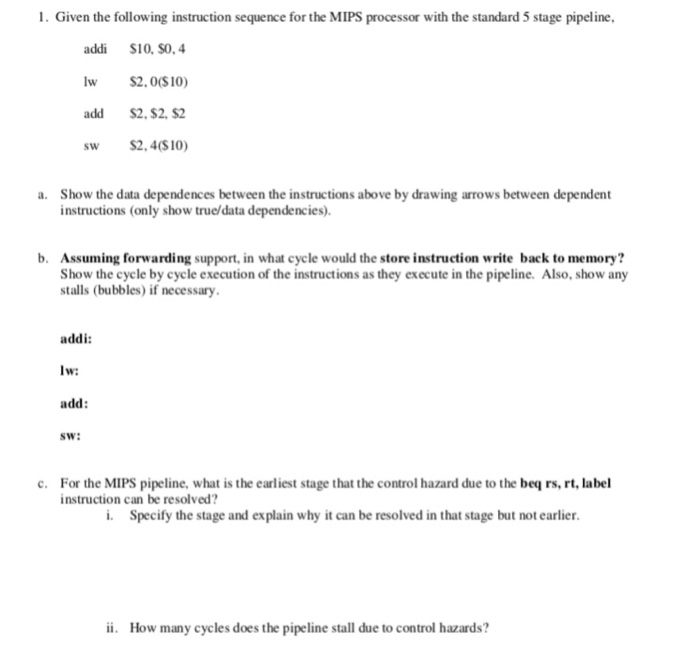1. Given the following instruction sequence for the MIPS processor with the standard 5 stage pipeline \$10, S0. 4 addi lw S2.0(S10) add sw S2,4(510) \$2, \$2, \$2 Show the data dependences between the instructions above by drawing arrows between dependent instructions (only show true/data dependencies). a. Assuming forwarding support, in what cycle would the store instruction write back to memory? Show the cycle by cycle execution of the instructions as they execute in the pipeline. Also, show any stalls...

• ### 1. Suppose we have a 5-stage pipeline CPU and run the following instructions: or \$tl, \$t2, \$t3 or...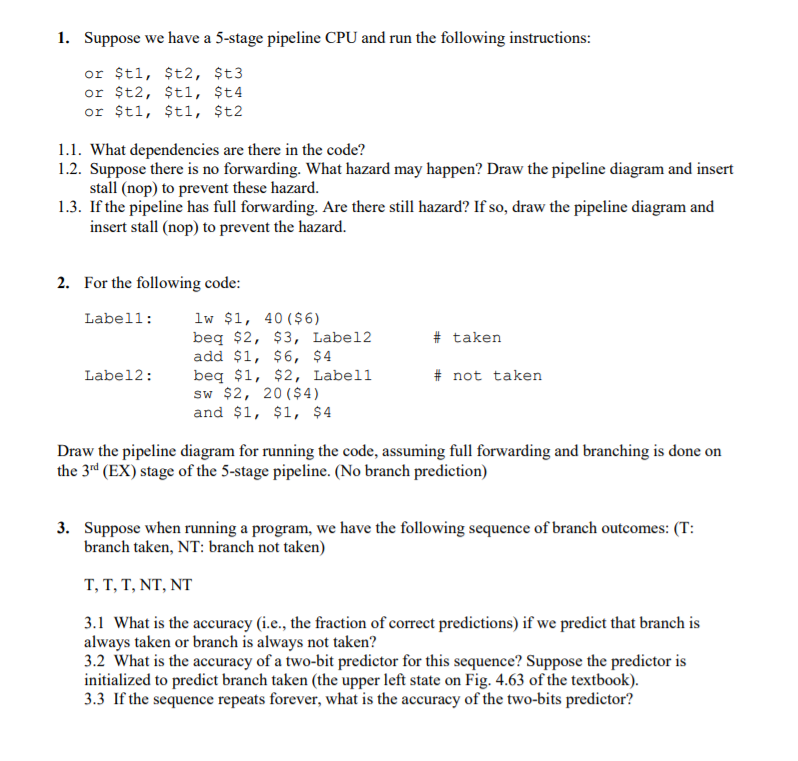1. Suppose we have a 5-stage pipeline CPU and run the following instructions: or \$tl, \$t2, \$t3 or \$t2, \$tl, \$t4 or \$tl, \$tl, \$t2 1.1. What dependencies are there in the code? 1.2. Suppose there is no forwarding. What hazard may happen? Draw the pipeline diagram and insert stall (nop) to prevent these hazard. 1.3. If the pipeline has full forwarding. Are there still hazard? If so, draw the pipeline diagram and insert stall (nop) to prevent the hazard....

• ### (60 points) The following instructions are executed on the 5-stage MIPS pipelined datapath. add r...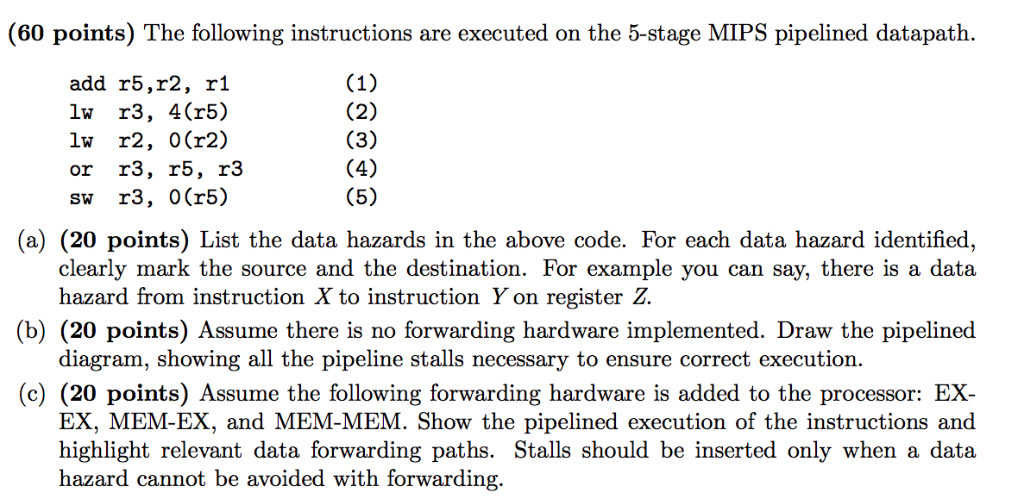Computer architecture help: (60 points) The following instructions are executed on the 5-stage MIPS pipelined datapath. add r5,r2, r1 lw r3, 4(r5) lw r2, 0(r2) or r3, r5, r3 sw r3, 0(r5) (a) (20 points) List the data hazards in the above code. For each data hazard identified, clearly mark the source and the destination. For example you can say, there is a data hazard from instruction X to instruction Y on register Z. (b) (20 points) Assume there is...

• ### We execute the following instruction sequence (data hazards may exhibit in the sequence) on a MIPS...

We execute the following instruction sequence (data hazards may exhibit in the sequence) on a MIPS 5-stage pipeline with forwarding and stall features implemented. Draw a graphical representation for the execution of the instruction sequence that clearly shows (1) forwarding path (if any); (2) stall cycles (if any); and (3) total cycles needed for the execution. lw R8, 0x0020(R10) add R9, R8, R10 sw R8, 0x0040(R10)

• ### 4. Suppose the MIPS code below is running on the pipeline processor we introduced in the...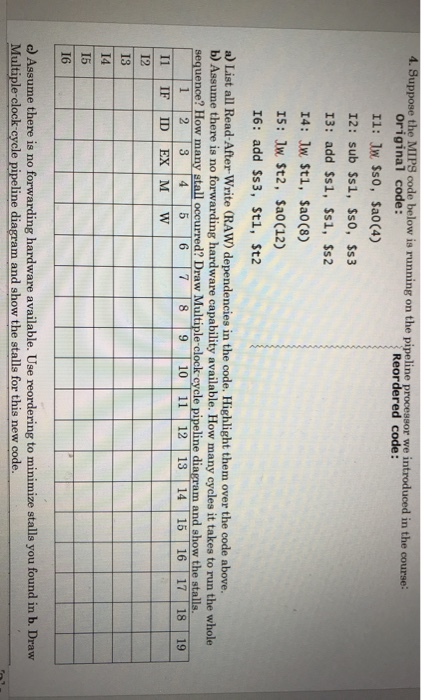4. Suppose the MIPS code below is running on the pipeline processor we introduced in the course: Original code: Reordered code: 11: Jw \$50, \$a0(4) 12: sub \$s1, \$50, \$s3 13: add \$81, \$si, \$s2 14: lw \$t1, \$a0(8) 15: Jw \$t2, \$a0(12) 16: add \$s3, \$t1, \$t2 a) List all Read-After-Write (RAW) dependencies in the code. Highlight them over the code above. b) Assume there is no forwarding hardware capability available. How many cycles it takes to run the...

• ### The following MIPS program is to be run on a MIPS pipeline processor of 5 stages...

The following MIPS program is to be run on a MIPS pipeline processor of 5 stages (IF-ID-EX-MEM-WB). Work out and diagram the optimal pipeline schedule using full forwarding from EX or MEM stages to any other stage, Draw the pipeline execution diagram for this code and then compute the pipeline CPI: addi \$t6, \$t6, 10 sub \$t5, \$t6, \$t4 srl \$t5, \$t5, 2 sw \$t5, 20(\$t5) lw \$t2, 0(\$t6) add \$t7, \$t2, \$t3 beq \$t5, \$t7, End

• ### Question 1: Problems in this exercise refer to the following sequence of instructions : LW \$5,...

Question 1: Problems in this exercise refer to the following sequence of instructions : LW \$5, -16(\$5) SW \$5, -16(\$5) ADD \$5, \$5, \$5 A) Indicate dependences and their type. (40 Points) B) Assume there is not forwarding in this pipeline processor, indicate hazards and add NOP instructions to eliminate them. C) Assume there is full forwarding, indicate hazards and add NOP instructions to eliminate unresolved cases. The remaining problem in this exercise assumes the following clock cycle times: Without...

• ### Q4. (20 points) Consider the following sequence of instructions being processed on the 5-stage RISC-V pipelined...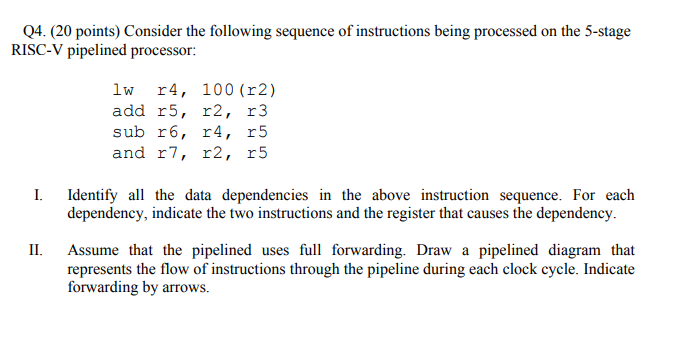Q4. (20 points) Consider the following sequence of instructions being processed on the 5-stage RISC-V pipelined processor: lw r4, 100 (r2) add r5, r2, r3 sub r6, r4, r5 and r7, r2, r5 I. Identify all the data dependencies in the above instruction sequence. For each dependency, indicate the two instructions and the register that causes the dependency. I Assume that the pipelined uses full forwarding. Draw a pipelined diagram that represents the flow of instructions through the pipeline during...

• ### How many clock cycles would be required to execute the following sequence of instructions? add \$t1,...

How many clock cycles would be required to execute the following sequence of instructions? add \$t1, \$t2, \$t3 #1 lw \$t3, 0(\$t1) #2 add \$t2, \$t2, \$t3 #3 add \$t3, \$t1, \$t2 #4